UPSC  >  Exercise 9.3 NCERT Solutions - Areas of Parallelograms and Triangles

# Exercise 9.3 NCERT Solutions - Areas of Parallelograms and Triangles - NCERT Textbooks (Class 6 to Class 12) - UPSC

Questions 1. In the figure, E is any point on median AD of a DABC.
Show that ar (ABE) = ar (ACE).
Solution:
We have a ΔABC such that AD is a median. ∴
ar (ΔABD) = ar (ΔADC)                ...(1)
[∵ A median divides the triangle into two triangles of equal areas.] Similarly, in DBEC, we have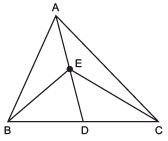ar (ΔBED) = ar (ΔDEC)                …(2)
Subtracting (2) from (1), we have
ar (ΔABD) - ar (ΔBED) = ar (ΔADC) - ar (ΔDEC)
⇒ ar (ΔABE) = ar (ΔACE).

Question 2. In a triangle ABC, E is the mid-point of median AD.
Show that ar (BED) =  ar (ABC).
Solution:
We have a ΔABC and its median AD.
Since, a median divides the triangle into two triangles equal in area.
∴ ar (ΔABD) = (1/2) ar (ΔABC)            …(1)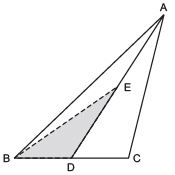Let us join B and E.
Now, in ΔABD, BE is a median. [∵ E is the mid-point of AD]
∴ ar (ΔBED) = (1/2)ar (ΔABD)

From (1) and (2), we have ar (ΔBED) = (1/2)[(1/2)]ar ( ABC) ]
⇒ ar (ΔBED) = (1/4)ar (ΔABC)

Question 3. Show that the diagonals of a parallelogram divide it into four triangles of equal area.
Solution:
We have a parallelogram ABCD such that its diagonals intersect at O.
∵ Diagonals of a parallelogram bisect each other.
∴ AO = OC and BO = OD Let us draw CE ⊥ BD.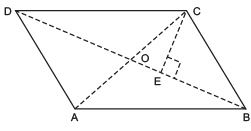Now, ar (ΔBOC) = (1/2) BO x CE
and ar (ΔDOC) = (1/2) OD x CE
Since, BO = OD ∴
ar (ΔBOC) = ar (ΔDOC)     …(1)
Similarly, ar (ΔAOD) = ar (ΔDOC)   …(2)
and ar (ΔAOB) = ar (BOC)        …(3)
From (1), (2) and (3), we have
ar (ΔAOB) = ar (ΔBOC)
= ar (ΔBOC) = ar (ΔAOD) Thus, the diagonals of a parallelogram divide it into four triangles of equal area.

Question 4. In figure, ABC and ABD are two triangles on the same base AB. If line segment CD is bisected by AB at O, show that ar (ABC) = ar (ABD).
Solution:
We have ΔABC and ΔABD are on the same base AB.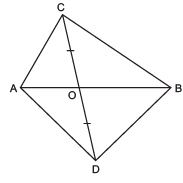∵ CD is bisected at O             [Given]
∴ AO = BO
Now, in ΔACD, AO is a median.
∴  ar (ΔOAC) = ar (ΔOAD)                  …(1)
Again, in DBCD, BO is a median.
∴  ar (ΔOBC) = ar (ΔODC)                     …(2)
Adding (1) and (2), we have ar (ΔOAC) + ar (ΔOBC)
= ar (ΔOAD) + ar (ΔODC)
⇒ ar (ΔABC) = ar (ΔABD)

Question 5. D, E and F are respectively the mid-points of the sides BC, CA and AB of a ΔABC. Show that
(i) BDEF is a parallelogram. (ii) ar (DEF) = (1/4)
ar (ABC)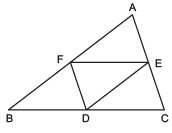(iii) ar (BDEF) = (1/2)ar (ABC)

Solution: We have: ΔABC such that the mid-points of BC, CA and AB are respectively D, E and F.
(i) To prove that BDEF is a parallelogram.
In ΔABC, E and F are the mid-points of AC and AB.
∴ EF || BC
⇒ EF || BD
Also EF =(1/2) BC
⇒ EF = BD           [∵ D is the mid-point of BC.]
∵ BDEF is a quadrilateral whose one pair of opposite sides is parallel and of equal lengths.
∴ BDEF is a parallelogram.

(ii) To prove that ar (DEF) = (1/4)ar (ABC).
We have proved that BDEF is a parallelogram.
Similarly, CDEF is a parallelogram and ΔEAF is a parallelogram.
Now, parallelogram BDEF and parallelogram CDEF are on the same base EF and between the same parallels BC and EF.
∴ ar (BDEF) = ar (CDEF)
⇒(1/2) ar (BDEF) = (1/2)ar (DEF)
⇒ ar (ΔBDF) = ar (ΔCDF) …(1)
[∵ Diagonal of a parallelogram divides it into two triangles of equal area]
Similarly, ar (ΔCDE) = ar (ΔDEF)         …(2)
ar (ΔAEF) = ar (ΔDEF)           …(3)
From (1), (2) and (3), we have
ar (ΔAEF) = ar (ΔFBD)
= ar (ΔDEF) = ar (ΔCDE)
Thus, ar (ΔABC) = ar (ΔAEF) + ar (ΔFBD) + ar (ΔDEF) + ar (ΔCDE)
= 4 ar (ΔDEF)
⇒ ar (ΔDEF) =(1/4) ar (ΔABC)

(iii) To prove that ar (BDEF) = (1/2)ar (ABC).
We have ar (BDEF)
= ar (ΔBDF) + ar (ΔDEF)
= ar (ΔDEF) + ar (ΔDEF)           [∵ ar (ΔDEF) = ar (ΔBDF)]
= 2 ar (ΔDEF)

= 2 [ (1/4)ar ( ABC)]
= 2 x (1/4)ar (ΔABC)
= (1/2)ar (ΔABC)
Thus, ar (BDEF) =(1/2)ar (ΔABC)

Question 6. In the figure, diagonals AC and BD of a quadrilateral ABCD intersect at O such that OB = OD. If AB = CD, then show that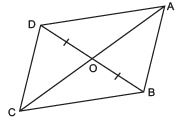(i) ar (DOC) = ar (AOB) (ii) ar (DCB) = ar (ACB) (iii) DA || CB or ABCD is a parallelogram.
Hint: From D and B, draw perpendiculars to AC.
Solution:
We have a quadrilateral ABCD whose diagonals AC and BD intersect at O. We also have that OB = OD
AB = CD
Let us draw DE ⊥ AC and BF ⊥ AC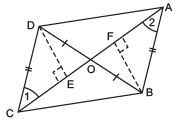(i) To prove that ar (ΔDOC) = ar (ΔAOB)
In ΔDEO and ΔBFO, we have
DO = BO             [Given]
–DOE = –BOF
[Vertically opposite angles]
–DEO = –BFO              [Each = 90º]
∴ ΔDEO ≌ ΔBFO               [ASA criteria]
⇒ DE = BF [c.p.c.t]
and ar (ΔDEO) = ar (ΔBFO) …(1)
[∵ Congruent triangle are equal in areas]
Now, in ΔDEC and ΔBFA, we have
–DEC = –BFA [Each = 90º]
DE = BF [Proved]
DC = BA [Given]
∴ ΔDEC ≌ ΔBFA                [RHS criteria]
⇒ ar (ΔDEC) = ar (ΔBFA)                     …(2)
Adding (1) and (2), we have ar (ΔDEO) + ar (ΔDEC) = ar (ΔBFO) + ar (ΔBFA)
⇒ ar (ΔDOC) = ar (ΔAOB)

(ii) To prove that ar (DCB) = ar (ACB)
∵ ar (ΔDOC) = ar (ΔAOB)            [Proved]
∴ Adding ar (ΔBOC), on both sides, we have
ar (ΔDOC) + ar (ΔBOC) = ar (ΔAOB) + ar (ΔBOC)
⇒ ar (ΔDCB) = ar (DACB)

(iii) To prove that DA || CB
∵ ΔDCB and ΔACB are on the same base CB and having equal areas.
∴ They lie between the same parallels CB and DA.
⇒ CB || DA (or ABCD is a parallelogram)

Question 7. D and E are points on sides AB and AC respectively of ΔABC such that ar (DBC) = ar (EBC). Prove that DE || BC.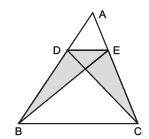Solution: We have ΔABC and points D and E are such that ar (DBC) = ar (EBC) Since, ΔDBC and ΔEBC are on the same base DE and having same area.
∴ They lie between the same parallels DE and BC. ⇒ DE || BC.

Question 8. XY is a line parallel to side BC of a triangle ABC. If BE || AC and CF || AB meet XY at E and F respectively, show that ar (ABE) = ar (ACF).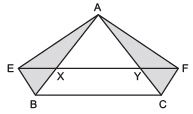Solution: We have a DABC such that  XY || BC, BE || AC and CF || AB.
Since, XY || BC and BE || CY
∴ BCYE is a parallelogram.
Now, the parallelogram BCYE and ΔABE are on the same base BE and between the same parallels BE and AC.

ar (ΔABE) = (1/2)ar (BCYE)            …(1)
Again, CF || AB                   [Given]
XY || BC                        [Given]
⇒ CF || AB and XF || BC
∴ BCFX is a parallelogram.
Now, ΔACF and parallelogram BCFX are on the same base CF and between the same parallels AB and FC.
∴ ar (ΔACF) = (1/2)ar (BCFX)               …(2)
Also parallelogram BCFX and parallelogram BCYE are on the same base BC and between the same parallels.
∴ ar (BCFX) = ar (BCYE)                 …(3)
From (1), (2) and (3) we get
ar (ΔABE) = ar(ΔACF)

Question 9. The side AB of a parallelogram, ABCD is produced to any point P. A line through A and parallel to CP meets CB produced at Q and then parallelogram PBQR is completed (see figure). Show that ar (ABCD) = ar (PBQR).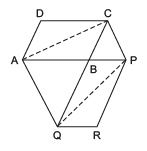Hint: Join AC and PQ. Now compare ar (ACQ) and ar (APQ).
Solution: We have a parallelogram ABCD. AB is produced to P. CB is produced to Q and PBQR is completed.
Let us join AC and PQ.
∵ ABCD is a parallelogram [Given] and AC is its diagonal.

ar (ΔABC) = (1/2)ar (parallelogram ABCD)               …(1)
∵ BQRP is a parallelogram [Given] and QP is its diagonal.
∴   ar (ΔBPQ) = (1/2)ar (parallelogram BQRP)              …(2)
Since ΔACQ and ΔAPQ are on the same base AQ and between the same parallels AQ and CP.
∴  ar (ΔACQ) = ar (ΔAPQ)                                [∵ Triangles on the same base and between the same parallels are equal.]
⇒ ar (ΔACQ)  - ar (ΔABQ) = ar (DAPQ) - ar (DABQ)               [Subtracting ar (ΔABQ) from both sides]
⇒ ar (ΔABC) = ar (ΔBPQ)                       …(3)
From (1), (2) and (3),
we get (1/2)ar (parallelogram ABCD) = (1/2)ar (parallelogram BQRP)

⇒ 2 [ (1/2)ar (parallelogram ABCD)] = 2 [(1/2)ar (parallelogram BQRP)]
⇒ ar (parallelogram ABCD) = ar (parallelogram BQRP)

Question 10. Diagonals AC and BD of a trapezium ABCD with AB || DC intersect each other at O. Prove that ar (AOD) = ar (BOC).
Solution:
We have a trapezium ABCD having AB || CD and its diagonals AD and BC are joined.
Since, triangles on the same base and between the same parallels have equal areas.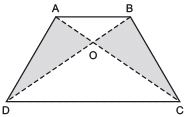∵ ΔABD and ΔABC are on the same base AB and between the same parallels AB and DC.
∴ ar (ΔABD) = ar (ΔABC)
Subtracting ar (ΔAOB) from both sides, we get
[ar (ΔABD) – ar (ΔAOB)] = [ar (ΔABC) – ar (ΔAOB)]
⇒ ar (ΔAOD) = ar (ΔBOC)

Question 11. In the figure, ABCDE is a pentagon. A line through B parallel to AC meets DC produced at F. Show that (i) ar (ACB) = ar (ACF) (ii) ar (AEDF) = ar (ABCDE)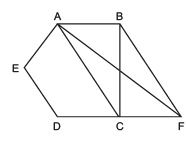Solution: We have a pentagon ABCDE in which BF || AC and DC is produced to F.

(i) To prove ar (ΔACB) = ar (ΔACF)
Since, the triangles between the same parallels and on the same base are equal in area.
∵ ΔACB and ΔACF are on the same base AC and between AC and BF.
∴ ar (ΔACB) = ar (ΔACF)

(ii) Since, ar (ΔACB) = ar (ΔACF) [Proved] Adding ar (AEDC) to both sides, we get
⇒ [ar (ΔACB) + ar (AEDC)] = [ar (ΔACF) + ar (AEDC)]
⇒ ar (AEDF) = ar (ABCDE)

Question 12. A villager Itwaari has a plot of land of the shape of a quadrilateral. The Gram Panchayat of the village decided to take over some portion of his plot from one of the corners to construct a Health Centre. Itwaari agrees to the above proposal with the condition that he should be given equal amount of land in lieu of his land adjoining his plot so as to form a triangular plot.
Explain how this proposal will be implemented.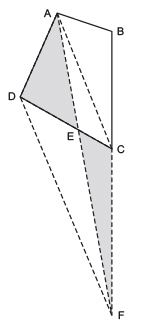Solution: We have a plot in the form of a quadrilateral ABCD.
Let us draw DF || AC and join A and F.
Now, ΔDAF and ΔDCF are on the same base DF and between the same parallels AC and DF.
∴  ar (ΔDAF) = ar (ΔDCF) Subtracting ar (ΔDEF) from both sides, we get
[ar (ΔDAF) - ar (ΔDEF)] = [ar (ΔDCF) - ar (ΔDEF)]
⇒ ar (ΔADE) = ar (ΔCEF)
The portion ΔADE can be taken over by the Gram Panchayat by adding the land (ΔCEF) to his (Itwaari) land so as to form a triangular plot,
i.e. ΔABF.
Let us prove that ar (ΔABF) = ar (quadrilateral ABCD), we have
ar (ΔCEF) = ar (ΔADE) [Proved] Adding ar (ABCE) to both sides, we get
[ar (ΔCEF) + ar (ABCE)] = [ar (ΔADE) + ar (ABCE)]
⇒ ar (ΔABF) = ar (quadrilateral ABCD)

Question 13. ABCD is a trapezium with AB || DC. A line parallel to AC intersects AB at X and BC at Y. Prove that ar (ADX) = ar (ACY).
Hint: Join CX.
Solution:
We have a trapezium ABCD such that AB || DC.
XY || AC meets AB at X and BC at Y.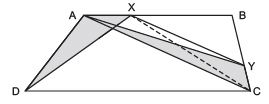Let us join CX.
∵ AB || DC [Given]
∴ ΔADX and ΔACX are on the same base AX and between the same parallels AB and DC.
∴  ar (ΔADX) = ar (ΔACX)             …(1)
∵ AC || XY [Given]

∴ ΔACX and ΔACY are on the same base AC and between the same parallels AC and XY.

∴  ar (ΔACX) = ar (ΔACY)           …(2)
From (1) and (2), we have

Question 14. In the figure, AP || BQ || CR. Prove that ar (AQC) = ar (PBR).
Solution:
We have AP || BQ || CR
Since, BQ || CR [Given]
∴ ΔBCQ and ΔBQR are on the same base BQ and between the same parallels BQ and CR.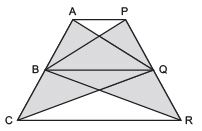⇒ ar (ΔBCQ) = ar (ΔBQR) …(1)
Again, AP || BQ [Given]
∵ ΔABQ and ΔPBQ are on the same base BQ and between the same parallels.
∴ ar (ΔABQ) = ar (ΔPBQ) …(2)
Adding (1) and (2), we have
[ar (ΔBCQ) + ar (ΔABQ)] = [ar (ΔBQR) + ar (ΔPBQ)]
⇒ ar (ΔAQC) = ar (ΔPBR)

Question 15. Diagonals AC and BD of a quadrilateral ABCD intersect at O in such a way that ar (AOD) = ar (BOC). Prove that ABCD is a trapezium.
Solution:
We have a quadrilateral ABCD and its diagonals AC and BD intersect at O such that ar (ΔAOD) = ar (ΔBOC).
Now, ar (ΔAOD) = ar (ΔBOC) [Given]
Adding ar (ΔAOB) to both sides, we have
[ar (AOD) + ar (AOB)] = [ar (BOC) + ar (AOB)]
⇒ ar (ΔABD) = ar (ΔABC)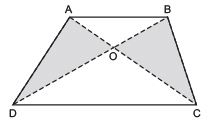But they are on the same base AB.
Since, the triangles on the same base and having equal area lie between the same parallels.
∴ AB || DC
Now, ABCD is a quadrilateral having a pair of opposite sides parallel.
∴ ABCD is a trapezium.

Question 16. In the figure, ar (DRC) = ar (DPC) and ar (BDP) = ar (ARC). Show that both the quadrilaterals ABCD and DCPR are trapeziums.
Solution:
We have ar (ΔDRC) = ar (ΔDPC)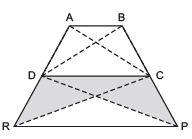[Given] But they are on the same base DC.
∴ ΔDRC and ΔDPC must lie between the same parallels.

⇒ DC || RP
⇒ One pair of opposite sides of quadrilateral DCPR is parallel.
∴ Quadrilateral DCPR is a trapezium.
Again, we have ar Δ(BDP) = ar Δ(ARC) [Given] .…(1)
Also ar (ΔDPC) = ar Δ(DRC) [Given] ….(2)
Subtracting (2) from (1),
we get [ar (ΔBDP) - ar (ΔDPC)] = [ar (ΔARC) - ar (ΔDRC)]
⇒ ar (ΔBDC) = ar (ΔADC) But they are on the same base DC.
∴ ΔBDC and ΔADC must lie between the same parallels.
⇒ AB || DC i.e. A pair of quadrilateral ABCD is parallel
∴ Quadrilateral ABCD is a trapezium.

The document Exercise 9.3 NCERT Solutions - Areas of Parallelograms and Triangles | NCERT Textbooks (Class 6 to Class 12) - UPSC is a part of the UPSC Course NCERT Textbooks (Class 6 to Class 12).
All you need of UPSC at this link: UPSC

## NCERT Textbooks (Class 6 to Class 12)

1402 docs|679 tests

## FAQs on Exercise 9.3 NCERT Solutions - Areas of Parallelograms and Triangles - NCERT Textbooks (Class 6 to Class 12) - UPSC

 1. What is the formula to find the area of a parallelogram?Ans. The formula to find the area of a parallelogram is base multiplied by the height, i.e., Area = base x height.
 2. How is the area of a triangle related to the area of a parallelogram?Ans. The area of a triangle is half the area of the parallelogram having the same base and height. In other words, if we have a parallelogram and draw a diagonal to form two triangles, the sum of the areas of these two triangles will be equal to the area of the parallelogram.
 3. Can we find the area of a parallelogram if only the lengths of its sides are given?Ans. No, we cannot find the area of a parallelogram if only the lengths of its sides are given. In addition to the lengths of the sides, we need either the measure of the included angle or the height of the parallelogram to find its area.
 4. What is the difference between a parallelogram and a rectangle?Ans. A parallelogram is a quadrilateral with opposite sides that are parallel, while a rectangle is a special type of parallelogram with all angles equal to 90 degrees. In other words, all rectangles are parallelograms, but not all parallelograms are rectangles.
 5. How can we find the area of a triangle if the lengths of its sides are given?Ans. If the lengths of the sides of a triangle are given, we can use Heron's formula to find its area. Heron's formula states that the area of a triangle with side lengths a, b, and c is given by the square root of s(s-a)(s-b)(s-c), where s is the semi-perimeter of the triangle (s = (a+b+c)/2).

## NCERT Textbooks (Class 6 to Class 12)

1402 docs|679 testsExplore Courses for UPSC exam### How to Prepare for UPSC

Read our guide to prepare for UPSC which is created by Toppers & the best Teachers
Signup to see your scores go up within 7 days! Learn & Practice with 1000+ FREE Notes, Videos & Tests.
10M+ students study on EduRev
Track your progress, build streaks, highlight & save important lessons and more!
Related Searches

,

,

,

,

,

,

,

,

,

,

,

,

,

,

,

,

,

,

,

,

,

;